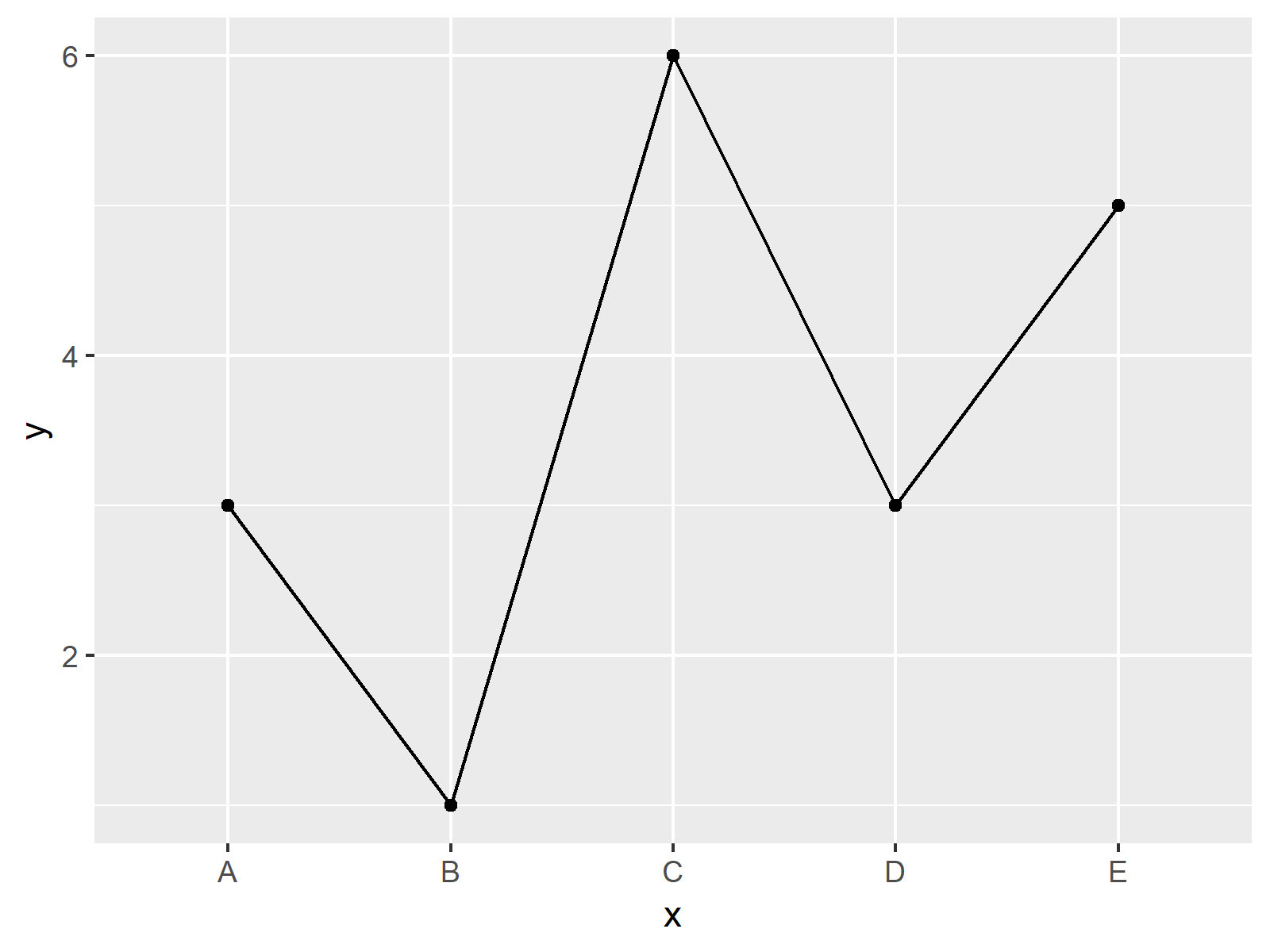# ggplot2 Warning – geom_path: Each group consists of only one observation. Do you need to adjust the group aesthetic?

In this article you’ll learn how to handle the warning message “geom_path: Each group consists of only one observation. Do you need to adjust the group aesthetic?” in R programming.

The table of content is structured as follows:

Let’s get started…

## Example Data

Consider the following example data:

```data <- data.frame(x = LETTERS[1:5], # Example data y = c(3, 1, 6, 3, 5)) data # Print data # x y # 1 A 3 # 2 B 1 # 3 C 6 # 4 D 3 # 5 E 5```

Have a look at the previous RStudio console output. It shows that our example data has two columns x and y. The variable x is a factor and the variable y has the numeric class.

## Example 1: Reproducing Warning – geom_path: Each group consists of only one observation. Do you need to adjust the group aesthetic?

The following R syntax shows how to replicate the warning message “geom_path: Each group consists of only one observation. Do you need to adjust the group aesthetic?”.

This warning appears when drawing plots with the ggplot2 package. Therefore, we first need to install and load the ggplot2 package, if we want to use the corresponding functions:

```install.packages("ggplot2") # Install ggplot2 package library("ggplot2") # Load ggplot2```

Now, we may try to create a ggplot2 line plot as shown below:

```ggplot(data, aes(x, y)) + # Trying to draw line plot geom_point() + geom_line() # geom_path: Each group consists of only one observation. Do you need to adjust the group aesthetic?```As shown in Figure 1, the previous syntax created a scatterplot instead of a line plot. Furthermore, we received the warning message “geom_path: Each group consists of only one observation. Do you need to adjust the group aesthetic?”.

## Example 2: Fixing Warning – geom_path: Each group consists of only one observation. Do you need to adjust the group aesthetic?

The following R programming code shows how to fix the geom_path warning message that we have seen in Example 1. For this, we need to specify the group argument within the aes function to be equal to 1:

```ggplot(data, aes(x, y, group = 1)) + # Specifying group = 1 geom_point() + geom_line()```Figure 2 shows the output of the previous R code – A line chart created by the ggplot2 package. Looks good!

## Video, Further Resources & Summary

Would you like to know more about warnings and errors in R? Then you may have a look at the following video of my YouTube channel. I explain the contents of this post in the video:

Besides the video, you might read the related tutorials of my website. I have published several articles about similar topics such as drawing numeric values with the ggplot2 package already:

Summary: In this tutorial, I illustrated how to handle the warning “geom_path: Each group consists of only one observation. Do you need to adjust the group aesthetic?” in R programming. Please tell me about it in the comments section below, in case you have any further comments and/or questions.

Subscribe to the Statistics Globe Newsletter

•Olivier
January 11, 2021 6:31 pm

I use excel data, which I import as .xlsx. When I apply the Group = 1 call, the error message does disappear, but also my first x-data point suddenly moves to behind the last x-data point. So my data is:

Frequency cells
5 kHz 14
10 kHz 18
20 kHz 17
30 kHz 19
40 kHz 18

So 5 rows and two columns. In the graph, the 5 kHz – 14 cells data point is plotted behind the 40 kHz, which is not what I want. Why is this?

Thanks!

• Hey Olivier,

I assume that this is because ggplot2 automatically orders your data alphabetically. You can reorder your data by releveling the factor levels of your data. Below, you can find a few tutorials that how to do this:

I hope this helps!

Joachim

•Oscar Acuña
May 28, 2021 10:49 pm

Hello
The fix is very easy, thanks for your excellent blog! You save me
Regards